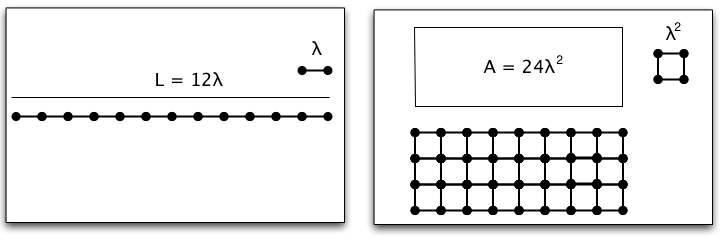• If you are citizen of an European Union member nation, you may not use this service unless you are at least 16 years old.

• Browse and search Google Drive and Gmail attachments (plus Dropbox and Slack files) with a unified tool for working with your cloud files. Try Dokkio (from the makers of PBworks) for free. Now available on the web, Mac, Windows, and as a Chrome extension!

View

# Scaling and functional dependence

last edited by 9 years, 11 months ago

## The Structure of the Way We See Reality: Functional dependence

In our pages on dimensional analysis, we have discussed it as what's called a passive transformation. That is, when we change a unit the physics system doesn't change, but the number we assign to it does. For different kinds of measurements, the number can change in a different way. This tells us something important about the kind of thing it is that we are talking about.

As an example, consider how we measure different kinds of physical space by comparing measurements of length and area. In both cases, we make a choice of a standard unit and count the number of times our unit fits in to get an assigned number. In the case of length, we use a small line segment, while in the case of area, we use a small box. Typically, we make the choice to make our area boxes squares with the side equal to our length segment. (This isn't absolutely necessary. Think about what we would have to do if we didn't do this.)When we fit a length, the number of times our unit length, λ, fits in depends linearly on the length we choose. (You can imagine λ as being, for example, 1 cm.)  When we fit square boxes of unit area, λ2, the number of boxes we fit in depends quadratically on the length we choose.

To see what we mean about changing scale, imagine that instead of a length λ, we choose a length scale half the size of our original scale, μ = λ/2. (This is like describing everything in terms of "demi-centimeters" instead of "centimeters") Our new measurements can be found algebraically in a straightforward way.

L = 12 λ = 12 (2 x λ/2) = 24 μ
A = 24 λ2 = 24 (2 x λ/2)2 = 24 x 4 (λ/2)2 = 96 μ2.

In both cases we have just replaced  λ by  λ/2.  But note that we have to change λ inside the square when we are working with the area. This shows that if we change our unit scale for length, the length and the area change in different ways.

This clarifies the reason why we cannot equate a length and an area. Even if the number assigned to them agrees in one system, it will not in any other.

### Active vs. Passive Transformations: Scaling

A viewpoint that makes these changes even more interesting is a switch from passive to active view. When we described why we wanted to make units match, we took a passive point of view. That is, we assumed that we had a fixed physical system and we were describing it in different units. We could flip this and take an active point of view. That is, we could assume that we have a fixed unit and we were changing our system. Thus, instead of saying that we switched to a unit half as big, we could say we switched to a length or area twice as big in all dimensions. Our argument then shows that doubling the size of our length, doubles its measure of length (duh!), but that doubling the size of our area (in all dimensions), quadruples its measure of area. This has powerful practical implications, especially in biology where one way evolution takes place is an organism keeps growing, scaling up its size. We have to understand scaling to see what the implications of this are. (For an example, see the Galileo scaling problem or the problem of the worm.)

How things change when we change some of the input descriptors is a general and powerful method both in biology and in advanced research physics. The general technique is associated with group theory: the mathematical tools for studying how things change. Darcy Thompson's 1917 book, On Growth and Form, provided an analysis for scaling of organisms with distortion that helped make sense of the role of development in evolution. Many books on biology include significant discussions of scaling, for example, C. J. Pennycuick, Newton Rules Biology: A physical approach to biological problems (Oxford U. Press, 1992). For an example in physics, general relativity was developed from Einstein's idea that the equations of physics should remain the same under any change of coordinates -- even complicated non-linear ones. Modern particle theories are constructed by choosing the descriptors and choosing groups associated with possible changes of the description of the system.

A fascinating modern research example of scaling arises from the observation that biological systems show scaling laws when they change their size. If you plot the log of the average number of heartbeats a minute for a given species vs. the log of the average mass of that species you get a straight line. That line has been known for almost 100 years, but there has been no explanation of it (on the order of our understanding of how the area changes as we change the length scale) until recently. One possible answer involves fractals. For an introduction to these ideas, check out the talk by Geoffrey West, one of the proposers of this explanation. A more modern analysis is given in J. Banavar, et al., "A general basis for quarter-power scaling in animals," PNAS 36, 15816-15820 (7 Sept 2010).

Joe Redish 7/8/11